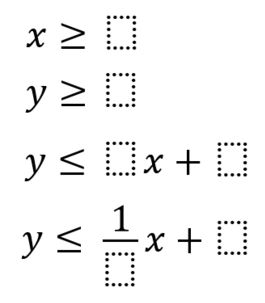# System of Inequalities

Directions: Fill each blank with a different integer such that the point (4,4) is within the solution region created by the constraints.### Hint

Where do the largest number need to go? Where do the smallest numbers need to go?
Use Desmos to graph each inequality.

There are various solutions. One possible solution is:
x >= 2
y >= 3
y <= -1/8x + 7
y <= -1x + 9

Source: Erick Lee

## Systems of Inequalities 2

Directions: Using the integers −9 to 9 at most one time each, place an integer …